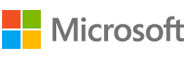New update is available. Click here to update.

# Euler’s Totient Function

Easy0/40
15 mins
90 %4 upvotes## Problem Statement

#### You are given an integer 'N'. Your task is to count the number of integers between 1 and 'N' both inclusive which are coprime to 'N'.

##### Note:
``````Two numbers are coprime if their greatest common divisor(GCD) is 1.

Here, 1 is considered to be coprime to any number.
``````
##### For Example:
``````If the given integer is 9, then the answer would be 6 Because there are six numbers between 1 and 9 both inclusive which are coprime to 9 i.e 1, 2, 4, 5, 7, and 8.
``````
Detailed explanation ( Input/output format, Notes, Images )
##### Constraints:
``````1 <= T <= 100
1 <= N <= 10 ^ 9

Time Limit: 1 sec.
``````
##### Sample Input 1:
``````2
1
4
``````
##### Sample Output 1:
``````1
2
``````
##### Explanation for Sample 1:
``````For the first test case, there is only one number which is coprime to 1 i.e 1 itself.

For the second test case, there are only two numbers between 1 and 4(both inclusive) which are coprime to 4 i.e 1 and 3.
``````
##### Sample Input 2:
``````2
12
21
``````
##### Sample Output 2:
``````4
12
``````
##### Explanation for Sample 2:
``````For the first test case, there are four numbers between 1 and 12(both inclusive) which are coprime to 12 i.e 1, 5, 7,11.
``````AutocompleteConsole